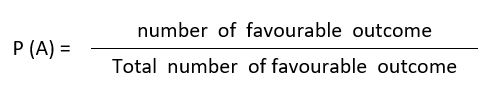Grab exciting probability worksheets for grade 6 with answers for your kids to learn how to calculate probability in a very practical way through simple and familiar real life circumstances.  In effect, these 6th grade probability questions with answers will offer kids strategic ways of reasoning, thus helpful to making adequate decisions every day in situations of chance.

##6th GRADE MATH PRINTABLES

### Best of FREE 6th Grade Math Worksheets Categories

As your kids engage in these interesting probability learning activities, their logical and mathematical thinking will be enhanced considering the fact that they’ll be dealing with uncertain situations, predicting events, deciding among different probabilities, problem solving etc.

It should equally be noted that kids will not only meet randomness in math, but equally in other areas, such as biology, economics, meteorology, social activities like games and sports, and in many other fields.

### Which methods are best to instil probabilistic thinking in kids?

Our 6th grade probability questions with answers are admirable tools with methods best to instil probabilistic thinking in kids.

As an important step in probability application, our exercises consist of real world phenomena, modelling random situations. This approach will provide much enthusiasm to your kids.

In addition, you’ll grab very simple basic probability formulas helpful to easily work out any given probability situation. For instance, the formula for calculating probability of an event is;•